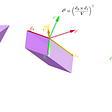# Visualizing The Product Rule

## Calculus: A Simple Proof

In today’s entry, we will take a look at the visualization of the product rule and gain a geometric understanding of what’s really going on.

Without further ado, let’s dive in!

Suppose we have a function H(x) = g(x)f(x).

--

--

--

## More from Intuition

We simplify science for you

## Gödel’s Completeness Theorem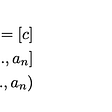## Calculus Series: A Slanted Revolution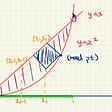## God is “normally distributed”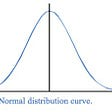## Let There Be… Electricity? Maxwell’s Equations: Explained## The Five Finger Curse## The 2 Parameter Binomial Discrete Distribution 4 Formulas## The Mean Value of A Function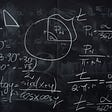19 | Math

## A Complex Numbers Question## The Fundamental Counting Principle## How To Really Avoid P-Value Hacking In Statistics?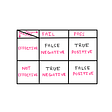## Glimpses of Dual Space